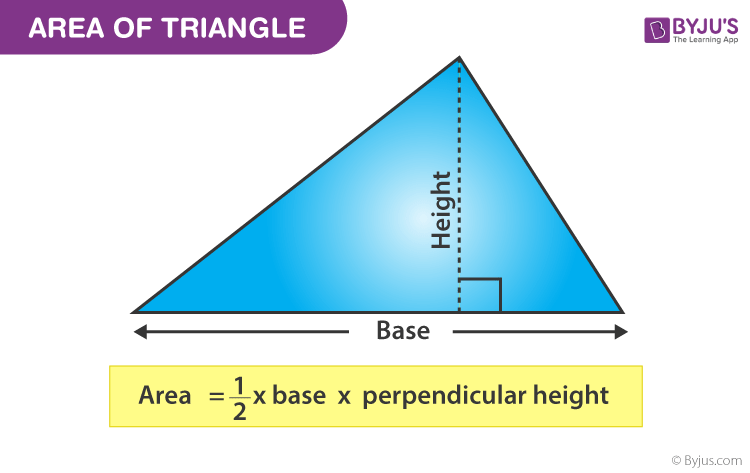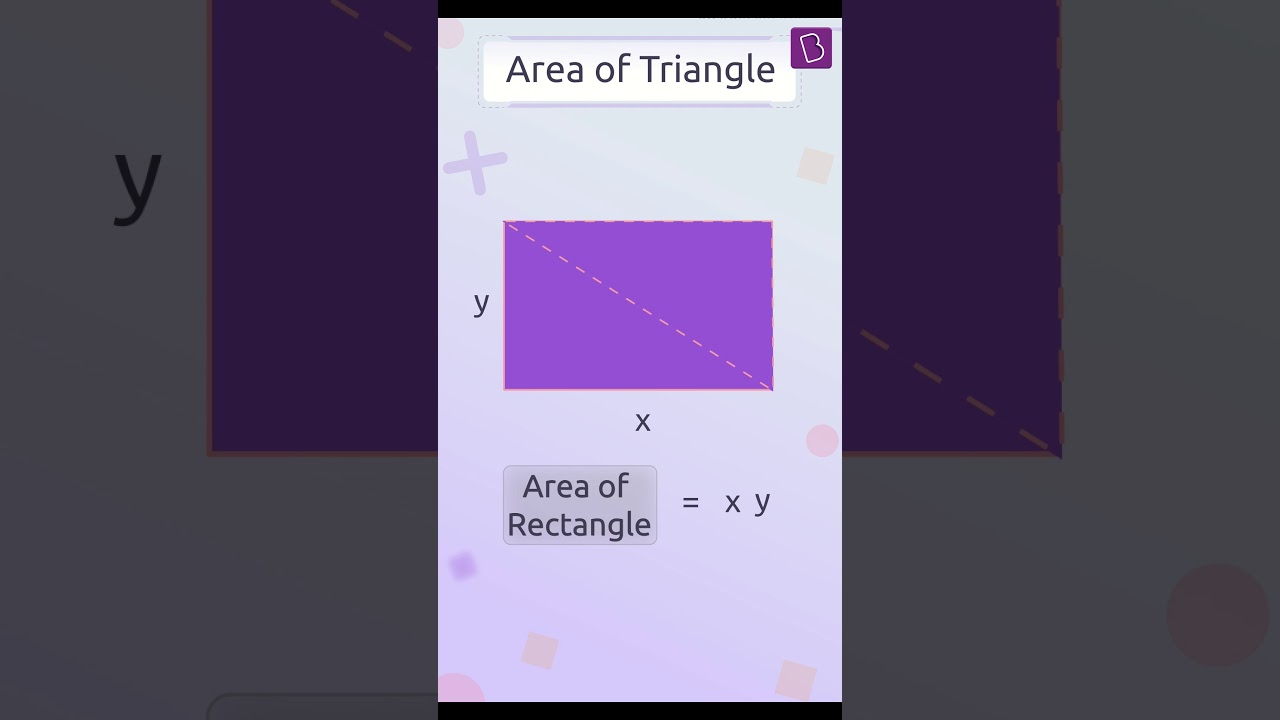# How to find the area of a triangle?

The area of a triangle is defined as the total region that is enclosed by the three sides of any particular triangle.

## Area of a Triangle Formula

The area of the triangle is given by the formula,

Area of a Triangle = A = ½ (b × h) square units

Where

• b is the base of the triangle
• h height of the triangle(1)(0)